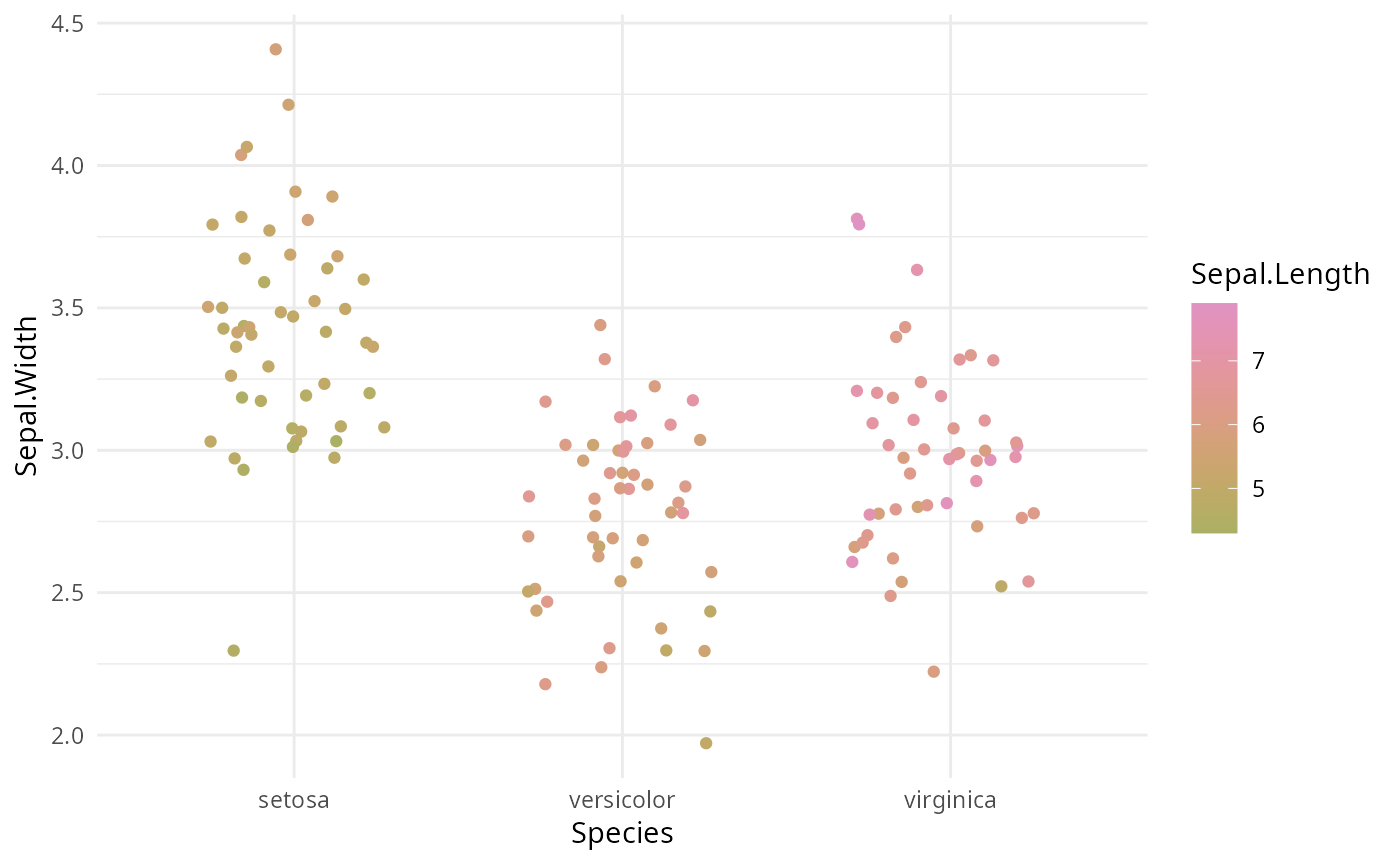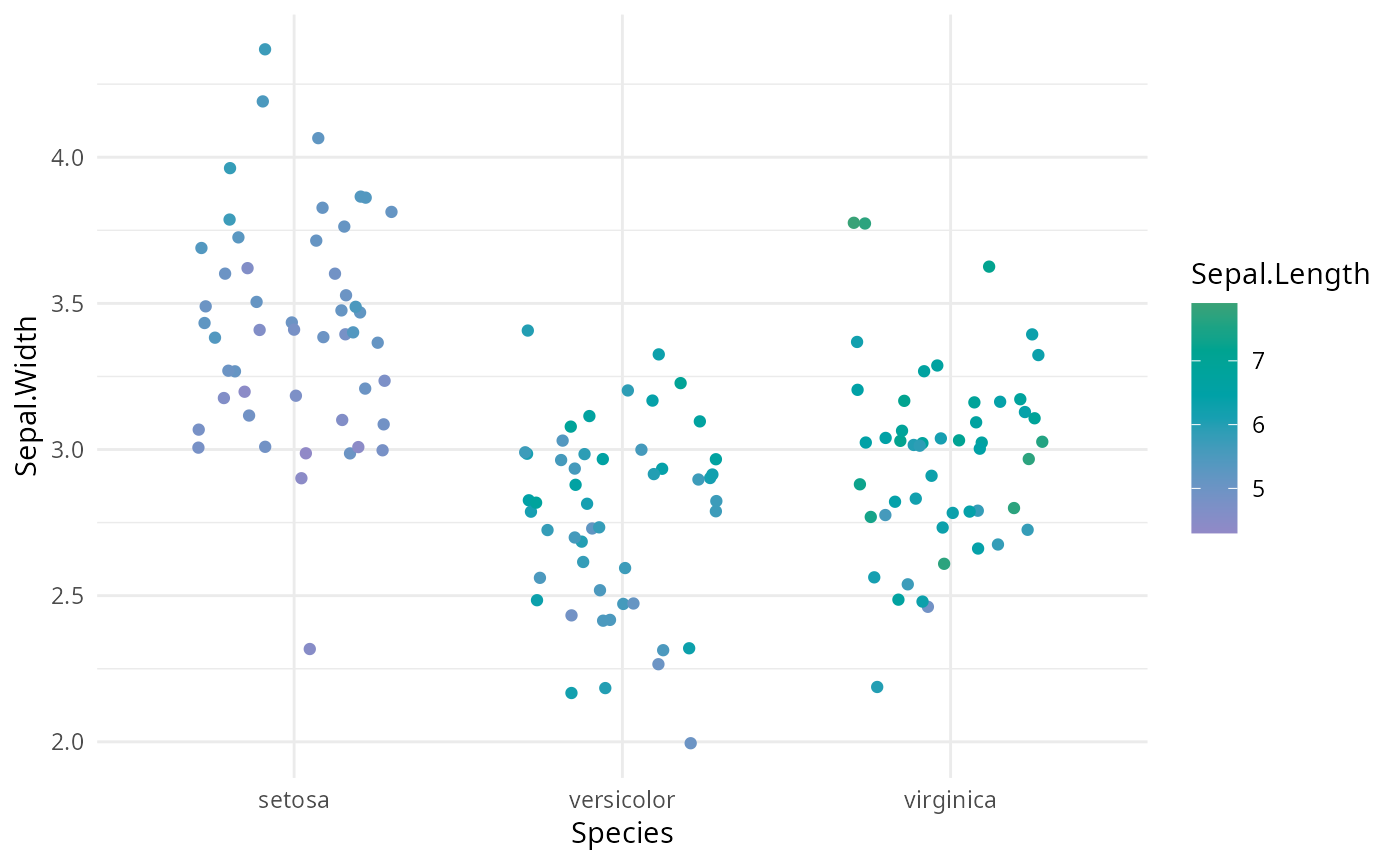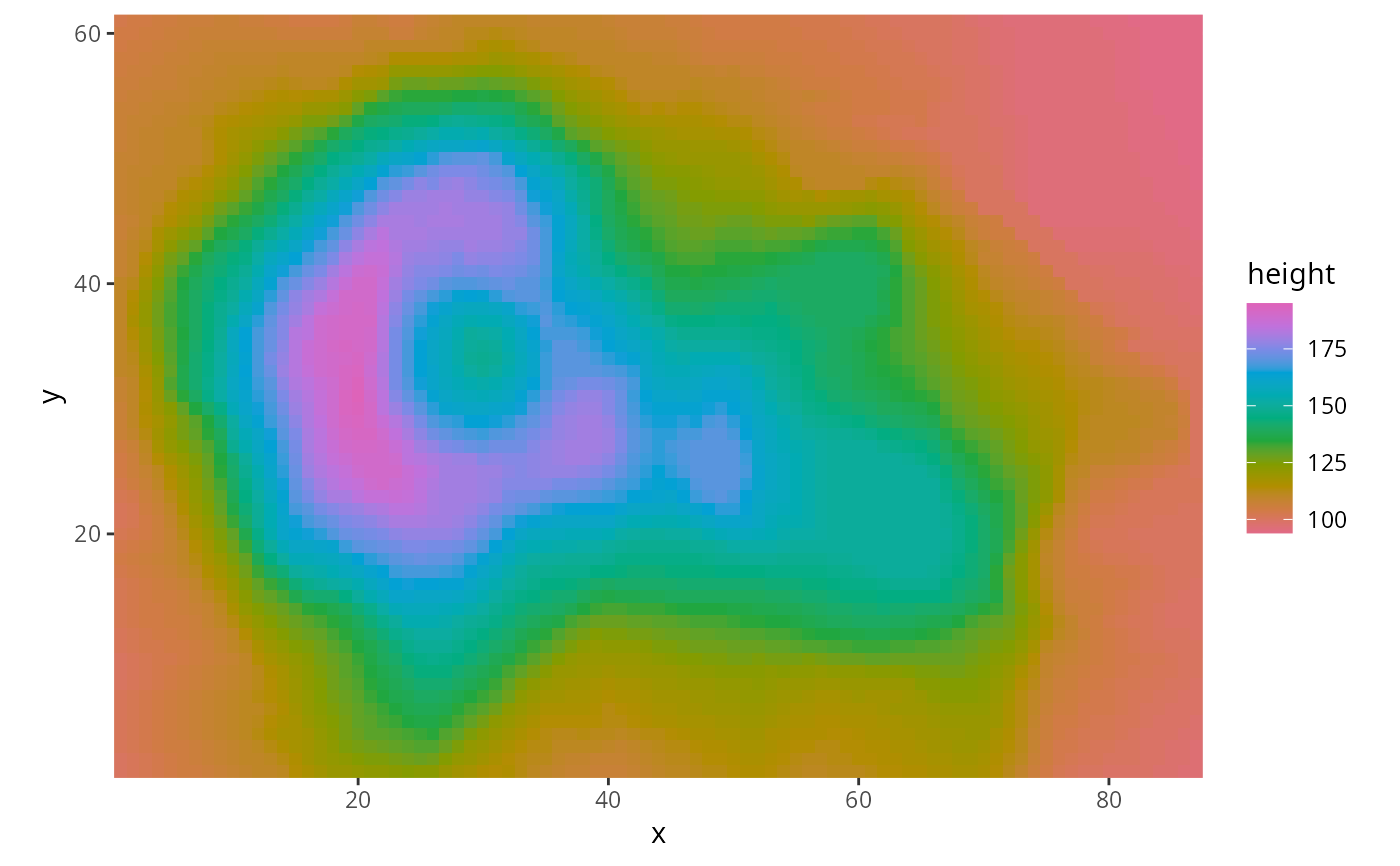Continuous ggplot2 color scales using the color palettes generated by qualitative_hcl. These scales are provided for completeness. It is not normally a good idea to color a continuous variable using a qualitative scale.

scale_colour_continuous_qualitative(
palette = NULL,
c1 = NULL,
l1 = NULL,
h1 = NULL,
h2 = NULL,
alpha = 1,
rev = FALSE,
begin = 0,
end = 1,
na.value = "grey50",
guide = "colourbar",
aesthetics = "colour",
n_interp = 11,
...
)

scale_color_continuous_qualitative(
palette = NULL,
c1 = NULL,
l1 = NULL,
h1 = NULL,
h2 = NULL,
alpha = 1,
rev = FALSE,
begin = 0,
end = 1,
na.value = "grey50",
guide = "colourbar",
aesthetics = "colour",
n_interp = 11,
...
)

scale_fill_continuous_qualitative(..., aesthetics = "fill")

## Arguments

palette

The name of the palette to be used. Run hcl_palettes(type = "qualitative") for available options.

c1

Chroma value, used for all colors in the scale.

l1

Luminance value, used for all colors in the scale.

h1

Beginning hue value.

h2

Ending hue value.

alpha

Numeric vector of values in the range [0, 1] for alpha transparency channel (0 means transparent and 1 means opaque).

rev

If TRUE, reverses the order of the colors in the color scale.

begin

Number in the range of [0, 1] indicating to which point in the color scale the smallest data value should be mapped.

end

Number in the range of [0, 1] indicating to which point in the color scale the largest data value should be mapped.

na.value

Color to be used for missing data points.

guide

Type of legend. Use "colourbar" for continuous color bar.

aesthetics

The ggplot2 aesthetics to which this scale should be applied.

n_interp

Number of discrete colors that should be used to interpolate the continuous color scale. 11 will work fine in most cases.

...

common continuous scale parameters: name, breaks, labels, and limits. See continuous_scale for more details.

## Details

If both a valid palette name and palette parameters are provided then the provided palette parameters overwrite the parameters in the named palette. This enables easy customization of named palettes.

## Examples

library("ggplot2")

# none of these examples are necessarily good ideas
gg <- ggplot(iris, aes(x = Species, y = Sepal.Width, color = Sepal.Length)) +
geom_jitter(width = 0.3) + theme_minimal()

gg + scale_color_continuous_qualitative(palette = "Warm")gg + scale_color_continuous_qualitative(palette = "Cold", l1 = 60)gg + scale_color_continuous_qualitative(palette = "Harmonic", rev = TRUE)nx = 87
ny = 61
df <- data.frame(height = c(volcano), x = rep(1:nx, ny), y = rep(1:ny, each = nx))
ggplot(df, aes(x, y, fill=height)) +
geom_raster() + scale_fill_continuous_qualitative(palette = "Dark 3") +
coord_fixed(expand = FALSE)